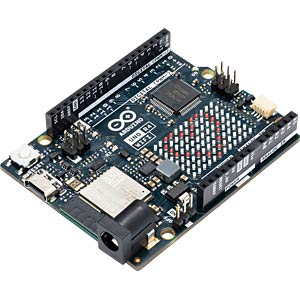In Arduino we can use the analogWrite(pin, value) method to create a PWM signal on the digital pins. The value parameter is used to change the duty cycle but the PWM frequency which is fixed at usually 490 Hz and can not be changed.Here is a simple example sketch that also works with the UNO R4:

``````void setup() {
pinMode(D2, OUTPUT);
// set 100%
analogWrite(D2,255)
}

void loop() {}
``````

Fortunately the Arduino UNO R4 provides some easy to use PwmOut class which provides more flexibility: The period needs to be defined in microseconds, so if you want to request a defined frequency in hz you can easily calculate this and there is a method to set the duty cycle using percent.

``````#include "pwm.h"

PwmOut pwm(D2);

void setup() {
//period 50us = 20000hz; pulse 0 us = 0%
pwm.begin(50,0.0);

// set 100%
pwm.pulse_perc(100.0);

}

void loop() {}
``````
Categories: Arduino

### 1 Comment#### Amrit · 12. September 2023 at 8:45

I want to know that how can I generate Complementary PWM signal using arduino r4, I need example for both using timers and the other way if there is any..